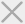• 安徽
• 北京
• 重庆
• 福建
• 广东
• 甘肃
• 黑龙江
• 湖北
• 河南
• 湖南
• 河北
• 吉林
• 江苏
• 江西
• 辽宁
• 内蒙古
• 上海
• 山西
• 山东
• 四川
• 陕西
• 天津
• 新疆
• 云南
• 浙江

• 哈尔滨

• 长春

• 沈阳

• 大连

• 北京

• 天津

• 石家庄

• 内蒙古

• 新疆

• 太原

• 济南

• 青岛

• 郑州

• 兰州

• 西安

• 南京

• 武汉

• 重庆

• 成都

• 上海

• 杭州

• 南昌

• 福州

• 厦门

• 长沙

• 广州

• 深圳

• 南宁

1. 雅思
2. 托福
3. 其它
4. 封闭学院

• 免费专区澳际学费在线支付平台

• iEduPay澳际人自己的支付平台

• 西联支付24小时到账

• 学易付支持所有教育部认证海外院校的在线缴费更多 +

更多 +
更多 +
更多 +
更多 +
更多 +
更多 +
更多 +

成功案例